Electric Fields and Electrostatics Electric fields are always

• Slides: 15Electric Fields and Electrostatics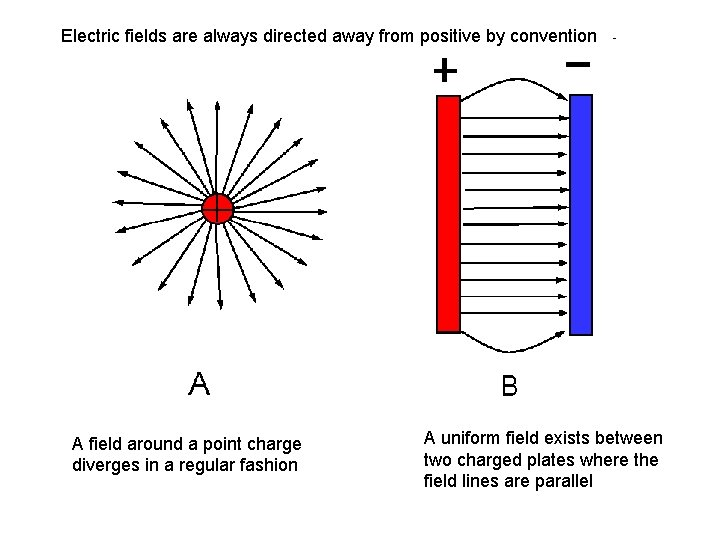Electric fields are always directed away from positive by convention A field around a point charge diverges in a regular fashion A uniform field exists between two charged plates where the field lines are parallelField Definition And Coulomb’s Law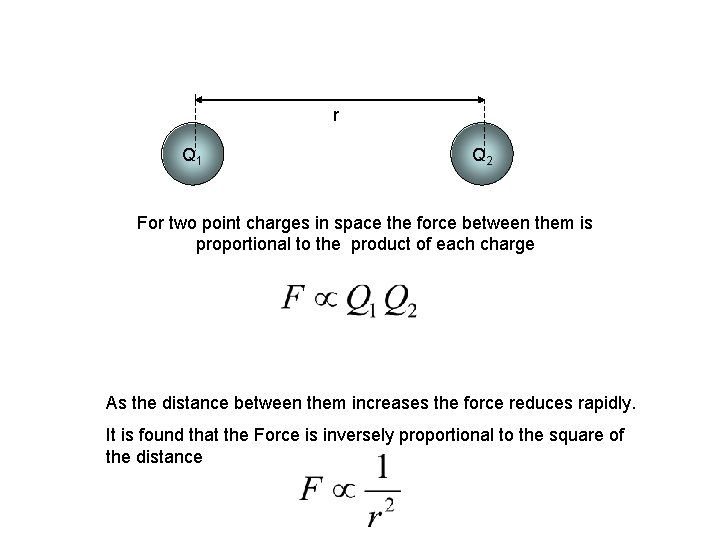r Q 1 Q Q 2 For two point charges in space the force between them is proportional to the product of each charge As the distance between them increases the force reduces rapidly. It is found that the Force is inversely proportional to the square of the distancer Q 1 Putting these two statements together we get Introducing a constant of proportionality Q Q 2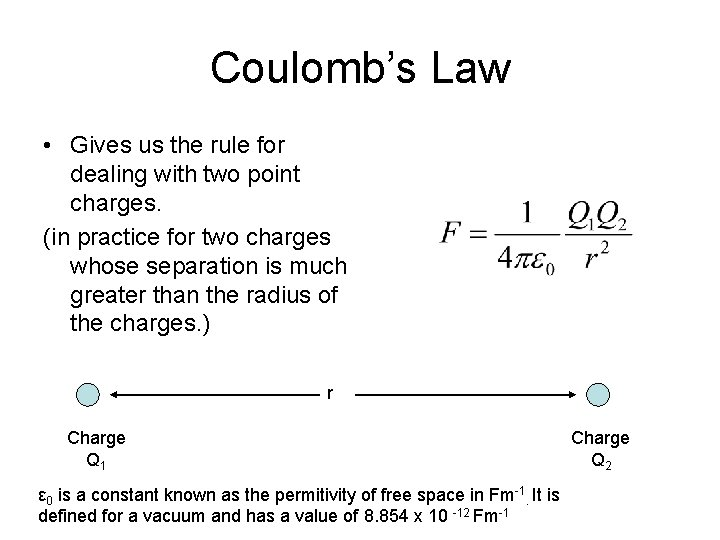Coulomb’s Law • Gives us the rule for dealing with two point charges. (in practice for two charges whose separation is much greater than the radius of the charges. ) r Charge Q 1 ε 0 is a constant known as the permitivity of free space in Fm-1. It is defined for a vacuum and has a value of 8. 854 x 10 -12 Fm-1 Charge Q 2A +++ B +++ Two metal spheres are given a charge of 3 x 10 -3 C and 4 x 10 -4 C each. Their distance of separation is 0. 5 m. What is the electrostatic force acting on A? What is the electrostatic force acting on B?The field strength of an electric field is defined by (Unit NC 1) That is the force exerted by the field on unit charge placed at that point. +1 C F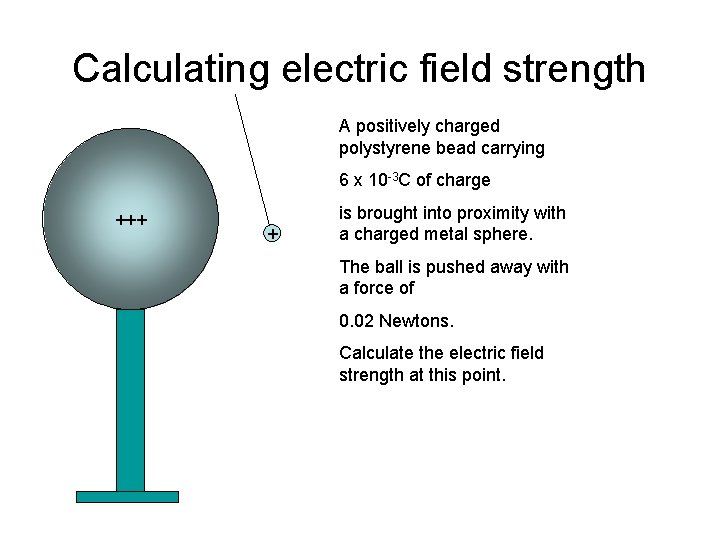Calculating electric field strength A positively charged polystyrene bead carrying 6 x 10 -3 C of charge +++ + is brought into proximity with a charged metal sphere. The ball is pushed away with a force of 0. 02 Newtons. Calculate the electric field strength at this point.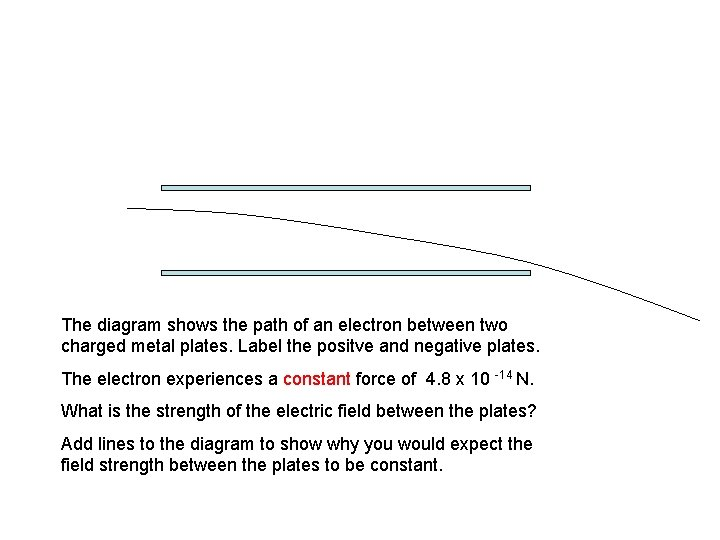The diagram shows the path of an electron between two charged metal plates. Label the positve and negative plates. The electron experiences a constant force of 4. 8 x 10 -14 N. What is the strength of the electric field between the plates? Add lines to the diagram to show why you would expect the field strength between the plates to be constant.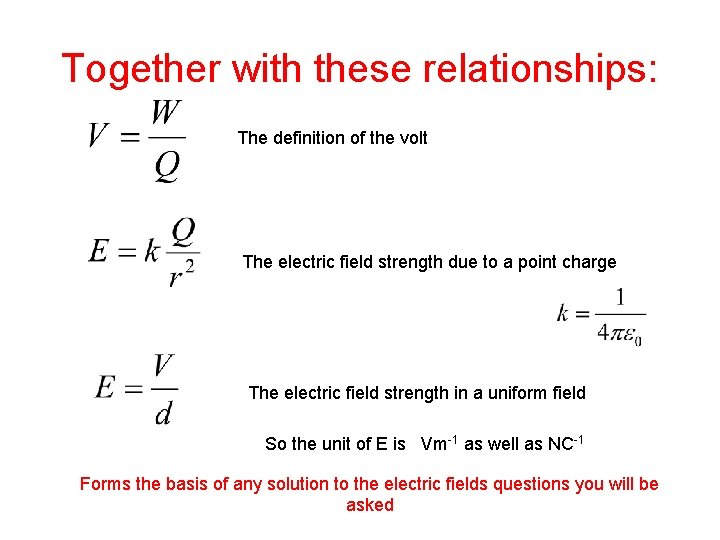Together with these relationships: The definition of the volt The electric field strength due to a point charge The electric field strength in a uniform field So the unit of E is Vm-1 as well as NC-1 Forms the basis of any solution to the electric fields questions you will be asked1. State Coulomb’s law for the electric force between two charged particles in free space. . . . . . . . . . . . . . . . . . . . . . . . . . . . . . . . . . . . (2) What are the base units of e 0 (the permittivity of free space)? . . . . . . . . . . . . . . . . . . . . . . . . . . . . . . . . . . . (2) (Total 4 marks)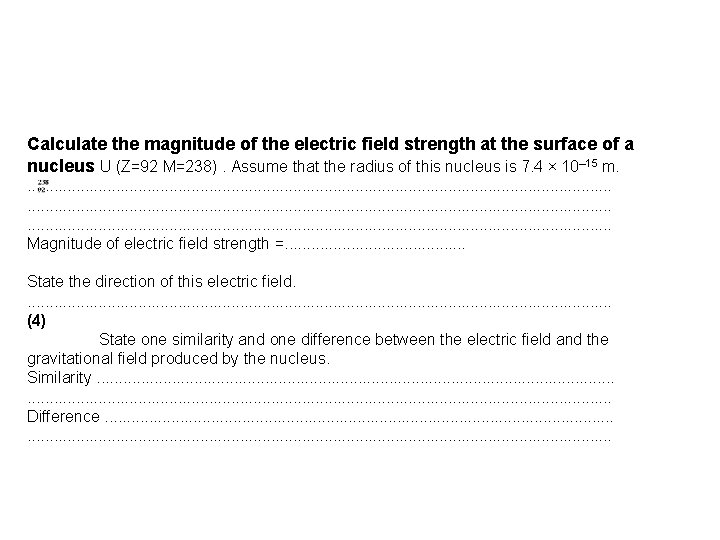Calculate the magnitude of the electric field strength at the surface of a nucleus U (Z=92 M=238). Assume that the radius of this nucleus is 7. 4 × 10– 15 m. . . . . . . . . . . . . . . . . . . . . . . . . Magnitude of electric field strength =. . . . . State the direction of this electric field. . . . . . . . . (4) State one similarity and one difference between the electric field and the gravitational field produced by the nucleus. Similarity. . . . . . . . . . . . . . . . Difference. . . . . . . . . . . . . . . .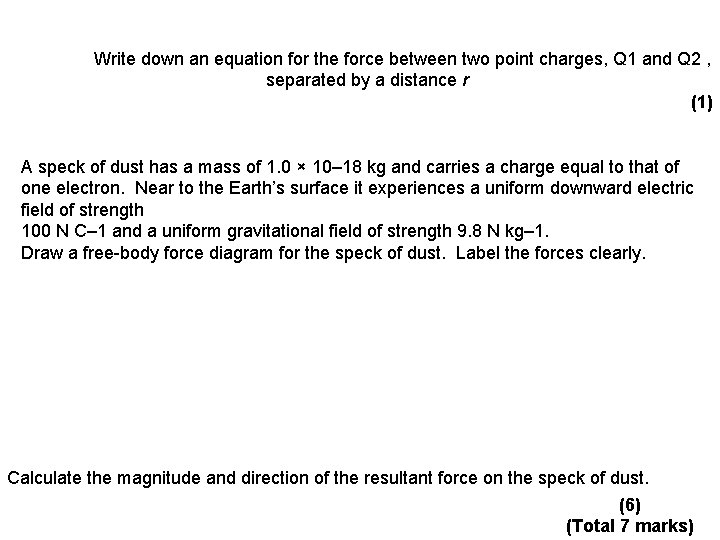Write down an equation for the force between two point charges, Q 1 and Q 2 , separated by a distance r (1) A speck of dust has a mass of 1. 0 × 10– 18 kg and carries a charge equal to that of one electron. Near to the Earth’s surface it experiences a uniform downward electric field of strength 100 N C– 1 and a uniform gravitational field of strength 9. 8 N kg– 1. Draw a free-body force diagram for the speck of dust. Label the forces clearly. Calculate the magnitude and direction of the resultant force on the speck of dust. (6) (Total 7 marks)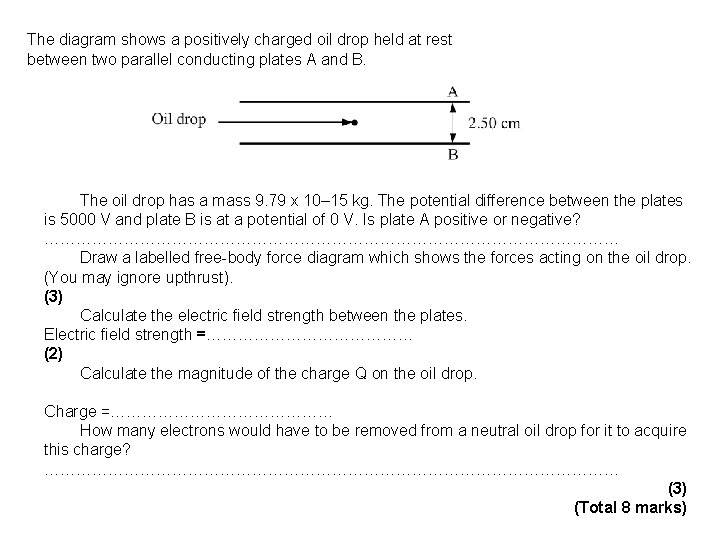The diagram shows a positively charged oil drop held at rest between two parallel conducting plates A and B. The oil drop has a mass 9. 79 x 10– 15 kg. The potential difference between the plates is 5000 V and plate B is at a potential of 0 V. Is plate A positive or negative? ……………………………………………… Draw a labelled free-body force diagram which shows the forces acting on the oil drop. (You may ignore upthrust). (3) Calculate the electric field strength between the plates. Electric field strength =………………… (2) Calculate the magnitude of the charge Q on the oil drop. Charge =………………… How many electrons would have to be removed from a neutral oil drop for it to acquire this charge? ……………………………………………… (3) (Total 8 marks)